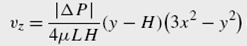### Create an Account

Home / Questions / An incompressible Newtonian fluid flows in the z direction through a straight duct of tria...

# An incompressible Newtonian fluid flows in the z direction through a straight duct of triangular cross sectional area bounded by the plane surfaces

An incompressible Newtonian fluid flows in the z-direction through a straight duct of triangular cross-sectional area, bounded by the plane surfaces H= √3 and =−√3 x. The velocity distribution under steady conditions is given byCalculate the volumetric flow rate.

Jul 25 2020 View more View LessSubscribe To Get Solution